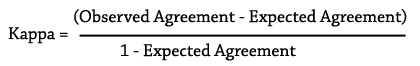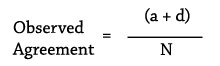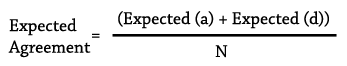# How to Calculate Kappa

What if the clinical diagnosis of Susser Syndrome was not considered a gold standard? In this case we cannot measure validity with sensitivity and specificity and we would instead measure reliability by calculating kappa. Kappa tells us the extent to which SussStat and the clinician agree with each other beyond what you might expect to see based on chance alone. The formula for Kappa is:We calculate observed agreement by calculating the frequency with which the two measurements agreed:We calculate expected agreement by first calculating the expected values of the cells in the 2×2 table using the marginal frequencies, then using those cell numbers to calculate the frequency with which the two measurements are expected to agree:### How to calculate expected cell frequencies:

Clinical Diagnosis
Positive Negative Total
SussStat Positive (a+b)(a+c)/N (a+b)(b+d)/N a+b
Negative (c+d)(a+c)/N (c+d)(b+d)/N c+d
a+c b+d N

When two measurements agree by chance only, kappa = 0. When the two measurements agree perfectly, kappa = 1.

Say instead of considering the Clinician rating of Susser Syndrome a gold standard, you wanted to see how well the lab test agreed with the clinician's categorization. Using the same 2×2 table as you used in Question 2, calculate Kappa. Scroll down for the answer.

### New table showing expected values:

Clinical Diagnosis
Positive Negative Total
SussStat Positive (130)(100)/(1000) = 13 (130)(900)/(1000) = 117 130
Negative (870)(100)/(1000) = 87 (870)(900)/(1000) = 783 870
100 900 1000

Observed agreement = (90 + 860) / 1000 = 0.950

Expected agreement = (13 + 783) / 1000 = 0.796

Kappa = (0.950 - 0.796) / (1-0.796) = 0.755

Interpretation : The SussStat test and the clinician had a probability of agreeing who had SusserSyndrome beyond chance of 0.755 (good agreement).